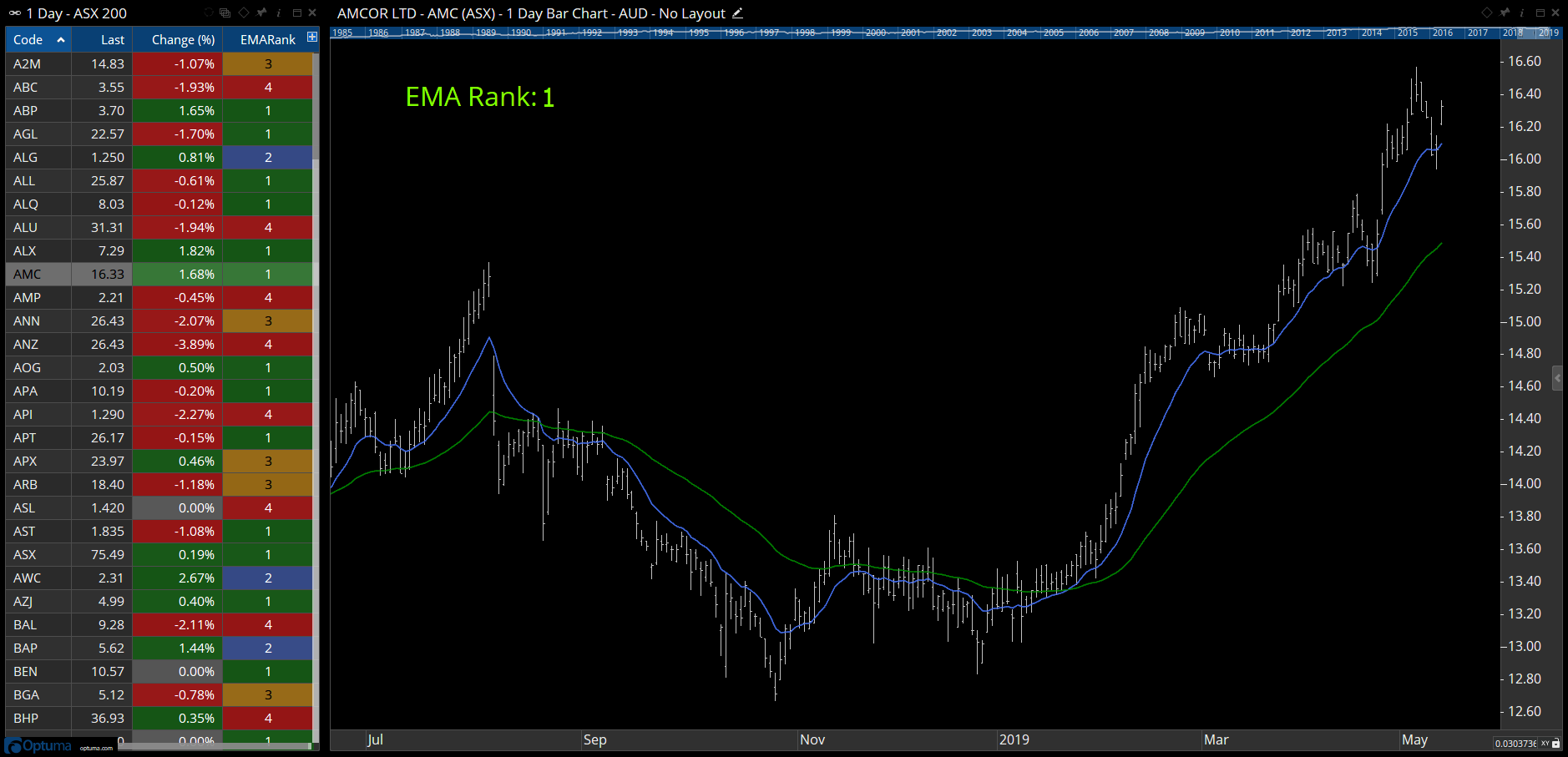# Conditional IF Statements

Author: Optuma Team Last updated: Nov 23, 2021 02:53

The IF() function allows you to create conditional logic statements, with the result being either true of false:

IF(formula, true value, false value)
For example, if the closing value is above the current 50MA use the closing value, but if it’s false use the current 50MA value:

IF (CLOSE()> MA(BARS=50),CLOSE(), MA(BARS=50))
Note that if the true and false values are not specified (i.e just the IF(CLOSE() > MA(BARS=50)) then it turns in to a true/false Boolean condition, with a value of 1 given to a true result, and zero for false. To have a green true or red false result in a watchlist column rather than a value simply use CLOSE() > MA(BARS=50) without using the IF function and a true or false result will show in the column.

Here’s an example of a nested IF statement for multiple values:

``````MA1 = MA(BARS=13, STYLE=Exponential, CALC=Close);
MA2 = MA(BARS=50, STYLE=Exponential, CALC=Close);
IF(CLOSE()>MA1, IF(CLOSE()>MA2, 1, 2), IF(MA2 IsUp, 3, 4))
``````

This will give the following values in a watchlist column, Chart Header, or Chart Element tool, based on the slope of the moving averages:

1 = close is above both the 13 Exponential MA and 50EMA, and both MAs are sloping up
2 = close is above the upward sloping 13EMA but below the downward sloping 50EMA
3 = close is below the downward sloping 13EMA and above the upward sloping 50EMA
**4 = close is below both the 13EMA and the 50EMA, **and both MAs are sloping down****